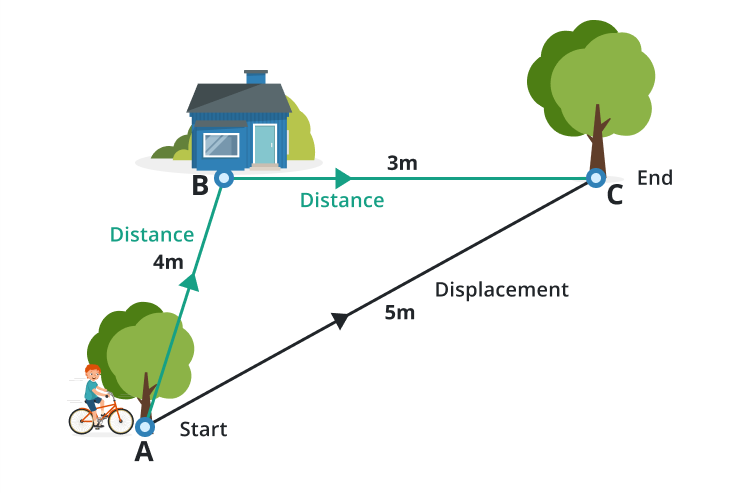# Displacement Calculator

Choose the quantity, fill the required input boxes, and press calculate button using displacement calculator

Formula:
s = 12 (vf + vi) t

Give Us Feedback

## Displacement Calculator

Displacement calculator is used to evaluate the displacement of an object using the time taken in its movements, and the initial & final velocity of the body in its movements.

## What is the displacement?

Displacement tells us the position or total distance covered by the object with respect to the initial position and final position. It is the distance traveled by an object between its starting and finishing points that is the shortest.Moreover, displacement is a vector quantity that includes the magnitude and direction. Its SI unit is a meter represented by the symbol “m”.

### Formula:

1. The mathematical formula of displacement is stated as.

`S = 1/2(vf + vi) × t`

1. To find the time of the object the formula becomes.

`t = 2S/vf + vi`

1. To find the final velocity of the object the formula becomes.

`vf = 2(S/t )- vi`

1. To find the initial velocity of the object the formula becomes.

`vi = 2(S/t) - vf`

Where,

• S = displacement of the object with respect to the position
• vf = object final velocity
• vi = object initial velocity
• t = time taken for the object to undergo displacement.

## How to find the displacement?

Example 1:

Evaluate the displacement of the object if the object moves with an initial velocity of 10 m/s in 20 seconds and finally comes in to rest with the speed of 30 m/s at the end.

Solution:

Step 1:

Write the data carefully from the above question.

S = displacement with respect to position =?,            vf = final velocity = 30

vi = initial velocity = 10,                                               t = time taken = 20

Step 2:

Write the formula of the displacement in detail.

`S = 1/2(vf + vi) × t`

Step 3:

Put all values in the above formula and simplify carefully.

vf = 30, vi = 10, t = 20

S = 1/2(vf + vi) × t

S = 1/2(30 + 10) × 20

S = 1/2(40) × 20

S = 20 × 20

`S = 400 m`

Example 2:

Evaluate the initial velocity of the object if the object covers 100 meters in 2 seconds and finally comes in to rest with the speed of 40 m/s at the end.

Solution:

Step 1:

Write the data carefully from the above question.

S = displacement with respect to position =100,       vf = final velocity = 40

vi = initial velocity = ?,                                                 t = time taken = 2

Step 2:

Write the formula of the initial velocity in detail.

`vi = 2(S/t) - vf`

Step 3:

Put all values in the above formula and simplify carefully.

S = 100, vf = 40, t = 2

vi = 2(S/t) - vf

vi = 2(100/2) - 40

vi = 2(50) – 40

vi = 100 - 40

`vi = 60 m/s`

Example 3:

Evaluate the final velocity of the object if the object covers 600m in 20 seconds and its initial velocity is 10 m/s.

Solution:

Step 1:

Write the data carefully from the above question.

S = displacement with respect to position = 600,      vf = final velocity = ?

vi = initial velocity = 10,                                               t = time taken = 20

Step 2:

Write the formula of the initial velocity in detail.

`vf = 2(S/t )- vi`

Step 3:

Put all values in the above formula and simplify carefully.

S = 600, vi = 10, t = 2o

vf = 2(S/t )- vi

vf = 2(600/20) - 10

vf = 2(30) – 10

vf = 60 - 10

`vf = 50 m/s`

### Math Tools